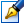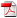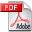##Covers of undirected graphs by convex sets

 Author: Buzatu Radu Degree: doctor of Speciality: 01.01.09 - Mathematical cybernetics and operation research Year: 2017 Scientific adviser: Sergiu Cataranciucdoctor habilitat, professor, Moldova State University Institution: Moldova State University

### Status

The thesis was presented on the 23 March, 2017
Approved by NCAA on the 31 May, 2017

### Abstract0.76 Mb / in romanian

### Thesis

CZU 519.833.58 Mb / in romanian
149 pages

### Keywords

undirected graph, d-convexity, d-convex set, metric segment, d-convex hull, optimization problem, d-convex cover, d-convex partition, NP-completeness, tree, algorithm

### Summary

The thesis has been elaborated in Moldova State University, Chisinau, 2017.

Thesis structure: The thesis is written in romanian language and contains an introduction, three chapters, general conclusions and recommendations, a bibliography of 115 titles, 122 pages of main text. The obtained results were published in 12 scientific papers.

The field of study: Graph theory

The aim of the research. The purpose of this PhD thesis is to study the problem of covering undirected graphs by d-convex sets. To achieve the purpose the following objectives are fixed: studying complexity of the problem of covering graphs by 2 p  d-convex sets; establishing conditions of existence of a d-convex set family covering an undirected graph; solving the problem of graph covering by nontrivial d-convex sets; developing algorithms for the problem of graph cover/partition by d-convex sets; determining the minimum/maximum d-convex cover number.

The scientific novelty and originality is reflected in obtaining theoretical and applied results which have supplemented and generalized known results related to graph cover by d-convex sets and in proving of NP-completness of the graph d-convex cover problem and some of its variations.

Important scientific problem solved in the research consists in proving of NP-completeness of nonoriented graph cover/partition by d-convex sets, which leads to the need to study conditions for existence of p  2 d-convex sets family, that cover/partition some graph classes for further implementation of methods and efficient algorithms for solving applied problems.

The theoretical significance of the research is determined by results associated with NP-completeness of graphs cover by two d-convex sets problem, which complements results in the field of study, obtained by other mathematicians . Also, it has been proven that the problems of general graphs cover by d-convex sets problem and graphs cover by nontrivial d-convex sets problem are NP-complete.

The applicative value of the paper consists in possibility of using obtained results for studying d-convex sets on discrete structures and in obtaining of algorithms for graphs cover/partition by d-convex sets problem, which can solve the investigated problem for practical applications, for example, for the task of clustering elements of a set with a binary relation defined over its elements.

The implementation of the scientific results. The results can be used in development of specialized courses for university students related to optimization problems on discrete structures. The developed algorithms are implemented as a library, written in C# programming language.